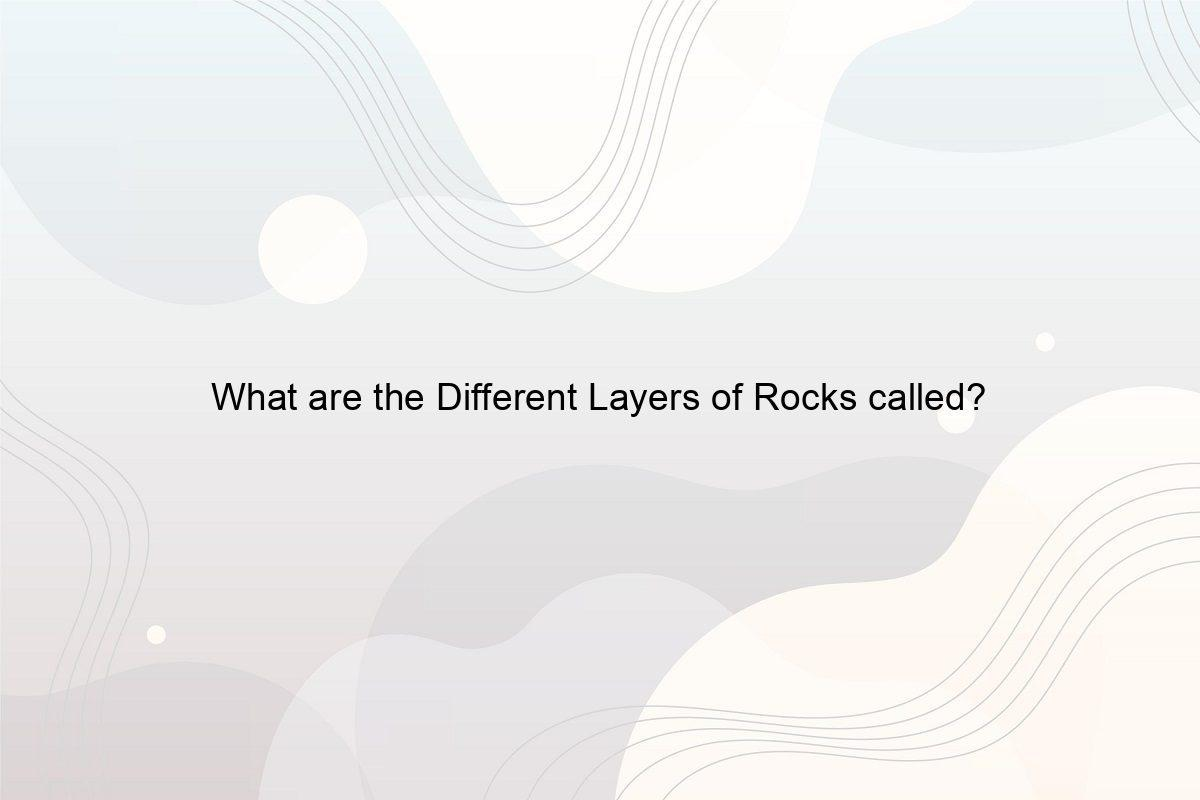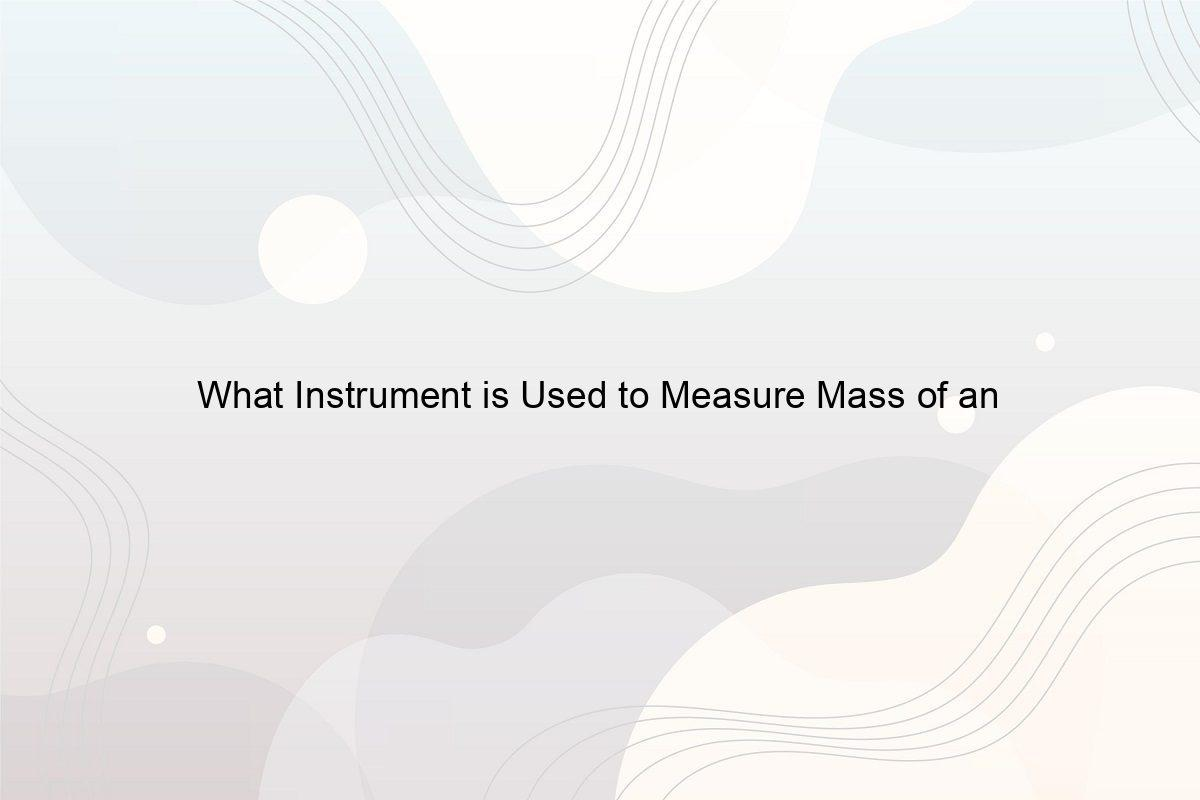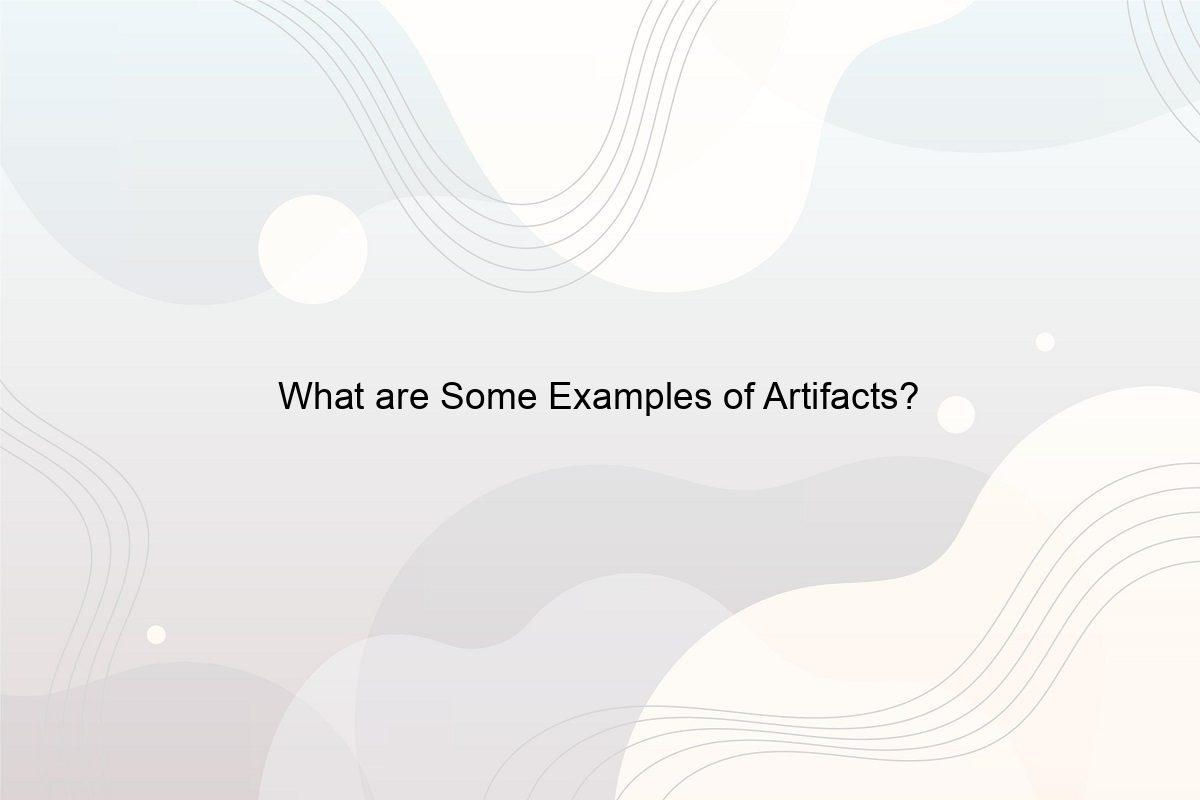﻿ How Big is a Centimetre Actual Size? - Speeli

# How Big is a Centimetre Actual Size?

What is a Centimeter? What is a Centimeter Example? How Big is a Centimeter in Real Life? How long is 1 cm on your Finger? What does Centimeter look like on a Ruler?

A centimeter is a unit of measurement often used to measure the length of an object. According to the metric system, a centimeter (denoted as cm) is equal to one-hundredth of a meter, or in simpler words, there are 100 centimeters in a meter. But do you ever wonder how big is a centimetre actual size? Let’s find out examples of a centimeter in real life, and measure 1 cm on your finger with a centimetre example. Scroll through the article and you will get all your answers.

### 1. What Size is Centimeter?

The metric unit system has various units of measurement to measure length, area, mass, and capacity. For measuring lengths, we have kilometers, millimeters, and centimeters; for measuring weight, we have grams and kilograms; for volume, we have liters, milliliters, etc. Each unit’s size can be determined or it can be converted into another unit. Conversion basically means shifting the decimal point. One just needs to keep in mind the metric prefix, and the conversion can easily be done by multiplying or dividing the number by the value of the prefix. (See How many cm in a Metre?)

We can write 1 cm = 1/100 m or 0.01 m.

Or, 1 cm = 10 millimetres.

### 2. What is Centimetre Example?

Are you trying to figure out a centimetre example? The most common centimeter example is a ruler. This measurement tool can measure small objects like notebooks, pencils, etc. Some other things that can be measured in centimeters are the waist size of pants, the length of a page, the sides of a triangle, measuring someone’s height, etc. (See What Instrument is Used to Measure Mass of an Object?)

### 3. How Big is a Centimeter in Real Life?

We know how big is a centimetre on a ruler and how big it looks on paper, although a centimeter in real life looks different when you look at it from a bigger perspective. 0.3937 inches make 1 cm. This means that 1 centimeter is less than half as much as an inch. Almost two-and-a-half centimeter forms one inch. So, a centimeter might not look that small on a piece of paper, but in real life, it is very tiny. So, how big is a centimetre actual size? Let’s find out. (See How long is 7 inches compared to an object?)

### 4. How Big is a Centimeter Actual Size?

Determining how big is a centimetre actual size is not that difficult. A centimeter’s actual size is exactly what you can see on the ruler that you use. It is equal to 10mm. You already know how to convert centimeters to meters, millimeters, and inches, in addition to the centimetre example. That is the actual size of a centimeter. That is how big a centimeter in real life is. (See How Big is 4 Inches?)

### 5. What Objects are 1 cm Long?

Image by Myriams-Fotos from Pixabay

How big is a centimetre actual size compared to everyday used items? Let’s list down some kinds of stuff that are 1 cm long that you usually see around us:

• The length of a staple.
• The width of a highlighter.
• The width of a standard notepad.
• The diameter of a belly button.
• The thickness of five CDs is stacked together.
• The nail bed of a lot of adult fingernails is approximately 1 cm.

Now, take a look around you and list down the objects you feel are exact or approximately 1 cm.

### 6. How Long is 1 cm on your Finger?

Take a ruler and measure how big is 1 cm on your finger. Our fingers are divided into three joints. You will realize that when you vertically place the ruler beside your finger, not even one entire joint is covered in 1 cm. Although the width of an average pinky finger is 1 cm, 1 cm does not cover a good length of the finger. (See How Deep is 10 Kilometers?)

### 7. What is 1cm Thick?

Are width and thickness the same? Or do length and thickness mean the same? Often getting confused? Let’s see, length, in simple words, means how long the object is. On the other hand, width means how thick the object is. So yes, width and thickness are the same. Some objects with a 1 cm thickness are the width of a pen or pencil, the width of a standard sticky notepad, the thickness of a pipe, etc. (See How many Metres is an Acre in the UK?)

### 8. How many cm is a Pinky Finger?

Image by Luisella Planeta Leoni LOVE PEACE 💛💙 from Pixabay

The pinky finger is the smallest finger on our hand. The length of the pinky finger varies from person to person, but you can easily measure it with a ruler. The average length of a pinky finger is somewhere around 5.6 cm and the width is 1 cm. (See Why is Your Small Finger Called a Pinky?)

### 9. What does Centimeters look like on a Ruler?

Do you also get confused about the two sides of the ruler? Let’s see which one of the sides is the centimeters. See along the long lines on the ruler, you’ll notice that these lines are numbered from 1 to 30. These are the centimeter marks. The distance between these numbers equals 1 cm. The smaller lines in between represent millimeters. (See How long is 8 inches compared to an object?)

### 10. How do you Measure Centimeters with your Hand?

Image by Elisa from Pixabay

How big is a centimetre actual size? You already have the answer. In ancient times, when rulers did not exist, people still found ways to measure objects. The most effective and widely used method was measuring with the hand. The hand has been used as the unit of length. For appropriate measuring, some of these measurements can be used.

• 1 inch(2.5 cm) = length of the first joint of your index finger.
• 2 inches(5 cm) = length of your thumb.
• 4 inches(10 cm) =  width of most people’s hands measured across their bottom knuckles (without the thumb).
• 6 inches(15 cm) = range from the tip of the thumb to the tip of the index finger (when a hand is spread wide).
• 9 inches (23 cm) = the distance between the tips of the thumb and the tips of the pinkies (when a hand is spread wide).
• 18 inches(46 cm) = range from the elbow to the tip of the middle finger.

In this article, we focused on various aspects of the metric unit of centimeters. We discussed centimetre example and learned how big is a centimeter in real life and how big is a centimetre actual size in a ruler. Hope the article was fun and knowledgeable for you. (See How Do You Convert 70 Inches to Feet?)

##### Related Posts## What are the Different Layers of Rocks called?

What are Rocks made up of? How many Layers does a Rock have? How do you determine the Order of Rock Layers?  Which Layer is made of Three Types of Rock?## What Instrument is Used to Measure Mass of an Object?

What is Mass? What is the SI unit of Mass? Which instrument is used to measure the weight of an Object? What are the five tools to measure Mass and Weight?